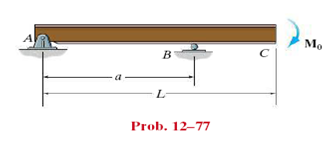### Determine the position a of roller support b

Assignment Help Civil Engineering
##### Reference no: EM131152448

Determine the position a of roller support B in terms of L so that deflection at end C is the same as the maximum deflection of region AB of the overhang beam. EI is constant.#### How long can the turbines produce 380 of power from the

How long can the turbines produce 380.0 MW of power from the release of the mass of stored water calculated above? Assume the water sustains a friction loss of 39.5 m2/s2 on t

#### Calculate the price of a nine-month american call option

Calculate the price of a nine-month American call option on corn futures when the current futures price is 198 cents, the strike price is 200 cents, the risk-free interest r

#### What is maximum unstretched cord to avoid reaching creek

Assume that the cord used in a bungee jump behaves like a linear spring when it is stretched with a spring constant of 80 lb/ft. A 180 lb student is going for a bungee jump

#### What is the resulting solids concentration

Raw primary sludge at a solids concentration of 3% is mixed with waste activated sludge at a solids concentration of 6,000 ppm. The flows are 20 and 25 gal/min, respectively.

#### Determine from curve the maximum flow rate

Determine from curve (a) critical depth (b) the maximum flow rate (c) the flow rate at a depth of 2.4 m and (d) the depths at which flow rate of 28.3 m^3/s may exist and the

#### Briefly describe requirements in design for interoperability

Define interoperability. Briefly describe the requirements in the design for interoperability. Describe some of the problems that could be encountered in meeting the objecti

#### Determine location of the instantaneous center of rotation

The center of gravity G of the 20-in. club is located 12 in. from the knob. knowing that at the instant, G has a velocity of 4 ft/s upwards and the club has an angular veloc

#### Determine the embedment depth and the anchor force

Determine the embedment depth and the anchor force per unit length of wall for the retaining wall shown in Figure P15.15 using the FMM. Assume the soil above the groundwater

### Write a Review